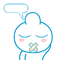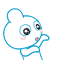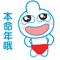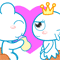# Python 中 arg,*args,**kwargs 简介

【摘要】本文记录有关 arg,*args,**kwargs 的相关知识。

1. `def test(arg,*args,**kwargs):`
2. `    `
3. `    print(arg,args,kwargs)`
4. `    `
5. `test(1,2,3,a = 4,b = 5,c = 6) `
6. `# 输出：1 (2, 3) {'a': 4, 'b': 5, 'c': 6}`

1. `def demo(a,b,*args,**kwargs):`
2. `    `
3. `    print(a,b,args,kwargs)`
4. `    `
5. `demo(1,2,3) `
6. `# 输出：1 2 (3,) {}`
7. `# 即：a = 1,b = 2,args = (3,),kwargs = {}`
8. `def demo(*args,**kwargs):`
9. `    `
10. `    print(args,kwargs)`
11. `    `
12. `demo(1,2,a = 10,b = 20) `
13. `# 输出：(1, 2) {'a': 10, 'b': 20}`
14. `# 即：args = (1,2) kwargs = {'a': 10, 'b': 20}`

arg：是单个变量

*args：是元组

**kwargs：则是比较常见的情况，如 a = 1,b = 2

*args,**kwargs 使用常见比较多的情况就是在 python 的装饰器中，因为参数的个数未知，所以运用的比较多。

0

0

 评论审核未开启 回复后邮件通知我• 浏览最多
• 评论最多